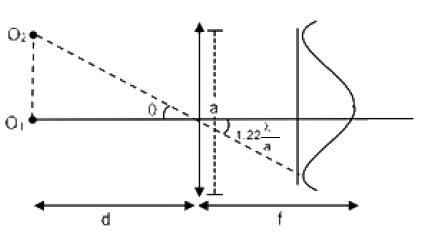# A telescope of aperture diameter 5 m is used to observe the moon from the earth

A telescope of aperture diameter 5 m is used to observe the moon from the earth. Distance between the moon and earth is 4 × 105 km. The minimum distance between two points on the moon’s surface which can be resolved using this telescope is close to (Wavelength of light is 5500 Å )

a) 60 m

b) 20 m

c) 600 m

d) 200 mMinimum angle for clear resolution,

θ= 1.22 (λ/a)

d = 1.22 (λ/a)d

r = 4×108 m

Aperture, a = 5

= 5500 A0

Distance = r d

= 4×108 ×1.22 (5500×10-10/5)

= 53.68

Nearest option is 60m.(0)(0)